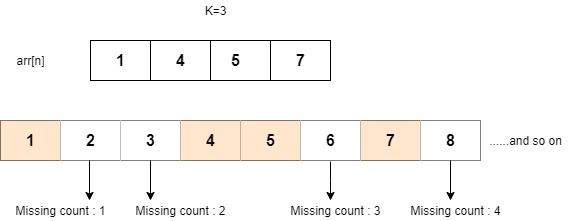New update is available. Click here to update.

# Kth Missing Element

Last Updated: 26 Nov, 2020
Difficulty: Moderate

## PROBLEM STATEMENT

#### Given an increasing sequence 'VEC', find the 'Kth' missing contiguous element in the given sequence starting from the leftmost element of the array.

##### Example :
``````Given 'VEC' : {1,4,5,7}
'K' : 3
````````````As shown in the above figure, numbers 2, 3, and 6 are missing. Since 6 is the third missing element, it is the required answer.
``````

#### Input Format :

``````The first line contains an integer ‘N’ denoting the number of elements in the array/list.

The second line contains ‘N’ space-separated integers denoting the elements of the array/list.

The third line contains an integer ‘K’ denoting the 'Kth' missing element.
``````

#### Output Format :

``````Print the 'Kth' missing contiguous element in the given sequence.
``````

#### Note :

``````You don't need to print anything, it has already been taken care of. Just implement the given function.
``````

``````Try to solve it in O(log(N)).
``````

#### Constraints :

``````1 <= N <= 10^4
1 <= K <= 10^9
-10^9 <= VEC[i] <= 10^9

Time Limit : 1 sec
``````

4 4 7 9 10 1## Approach 1

For each element check whether the current and next element is consecutive or not. If not, take the difference between the two and check till the difference is greater or equal to the given value of ‘K’. If the difference is greater, return current element - ’COUNT’.

Here is the algorithm :

1. Run a loop from 0 to ‘N’ - 2 (say, iterator ‘i’).
2. If ‘VEC[i] + 1’ is not equal to ‘VEC[i + 1]’, that is, elements are not consecutive, save their difference in a variable (say, ‘DIFFERENCE)’ and decrement by one, which will give the count of absent elements between the elements ‘VEC[i]’ and ‘VEC[i + 1]’.
• If the difference is lesser than ‘K’, deduct this difference from ‘K’. It means the missing element is not present between these 2 elements and the missing element count between these two elements is deducted
• Else if the difference is greater than or equal to ‘K’, it means the missing element exists between the elements ‘VEC[i]’ and ‘VEC[i + 1]’. So, ‘VEC[i]’ + ‘K’ will give the ‘Kth’ missing contiguous element in the given sequence starting from the leftmost element of the array. Turn ‘FLAG’ to ‘true’.
3. If ‘FLAG’ is true, return the answer calculated in step 2b.
4. Else, the answer will be greater than ‘VEC[n - 1]’, so return ‘VEC[n - 1]’ + ’K’.